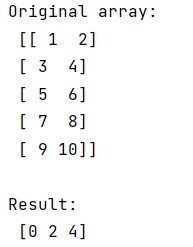# Find the row indexes of several values in a NumPy array

NumPy | Row indexes of several values: In this tutorial, we will learn how to find the row indexes of several values in a NumPy array in Python? By Pranit Sharma Last updated : April 20, 2023

## Problem Statement

Suppose that we are given a 2D numpy array and we need to find the row index of several values in this array.

For example, if we are given an array as:

```[[1,  2],
[3,  4],
[5,  6],
[7,  8],
[9,  10]]
```

And, we need to extract the indices of [1,2], [5,6] and [9,10].

## Finding the row indexes of several values

A memory-efficient approach is to find the row indexes of several values in a NumPy array, simply convert each row as linear index equivalents and then check if each element of an array is present in another by using the combination of np.in1d() and np.where() methods.

## Python program to find the row indexes of several values in a NumPy array

In this program, we have a 2D NumPy array and a set of values to be searched within the 2D array. We are finding the row indexes of these values, and printing the result.

```# Import numpy
import numpy as np

# Import pandas
import pandas as pd

# Creating an array
arr = np.array([[1,  2],
[3,  4],
[5,  6],
[7,  8],
[9,  10]])

# Display original array
print("Original array:\n",arr,"\n")

# Searching values
Searched_Values = np.array([[1, 2],
[5, 6],
[9, 10]])

# linear index
dims = arr.max(0)+1

# Multiple index
res = np.where(np.in1d(np.ravel_multi_index(arr.T,dims),np.ravel_multi_index(Searched_Values.T,dims)))

# Display result
print("Result:\n",res)
```

### Output